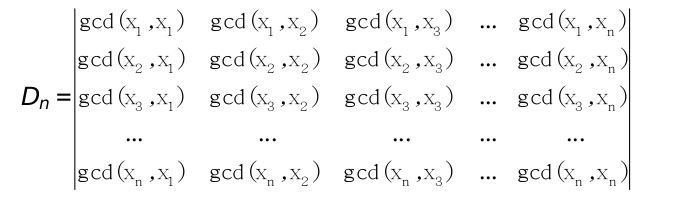# 2750. GCD Determinant

We say that a set S = {x1, x2, …, xn} is factor closed if for any xi ∈ S and any divisor d of xi we have d ∈ S. Let’s build a GCD matrix (S) = (sij), where sij = GCD(xi, xj) – the greatest common divisor of xi and xj. Given the factor closed set S, find the value of the determinant:### 输入格式

The input file contains several test cases. Each test case starts with an integer n (0 < n < 1000), that stands for the cardinality of S. The next line contains the numbers of S: x1, x2, …, xn. It is known that each xi is an integer, 0 < xi < 2*109. The input data set is correct and ends with an end of file.

### 输出格式

For each test case find and print the value Dn mod 1000000007.

### 样例

Input
2
1 2
3
1 3 9
4
1 2 3 6

Output
1
12
4


8 人解决，13 人已尝试。

8 份提交通过，共有 34 份提交。

6.9 EMB 奖励。